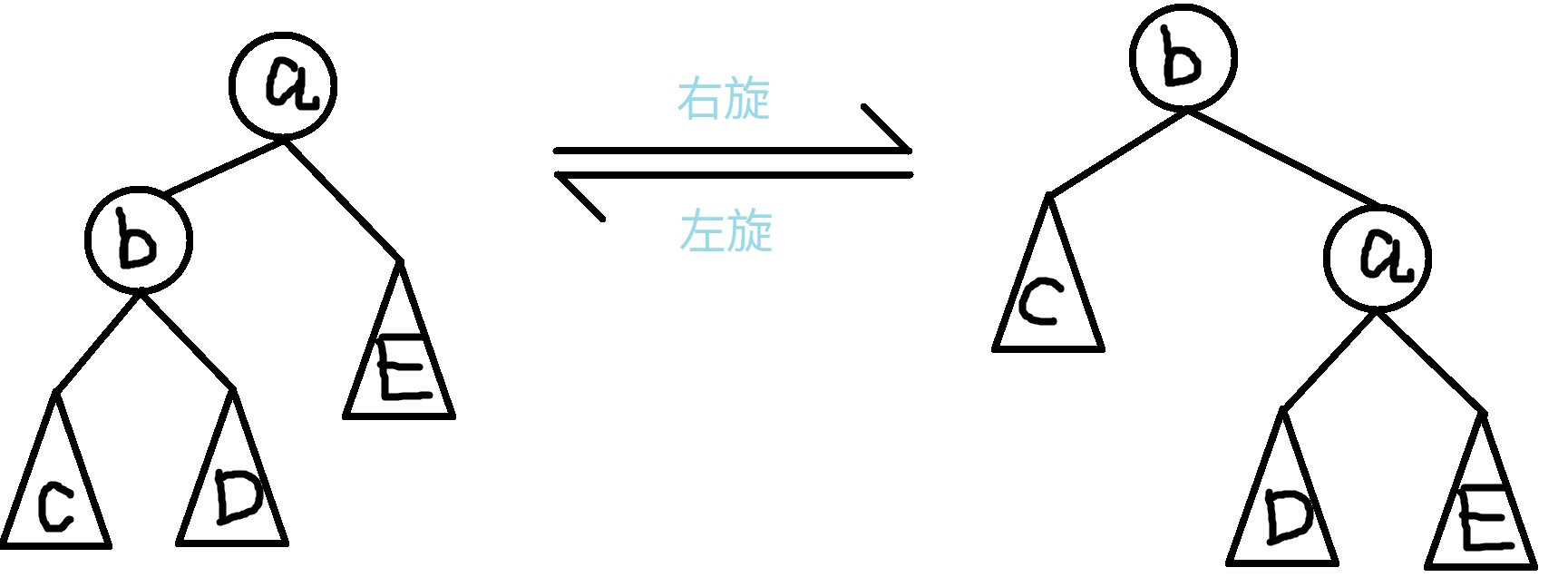• ChungZH's blog
• ## Treap 笔记

• @ 2022-8-20 10:27:58

Treap = Tree + Heap

## 二叉搜索树（BST）

• $x$ 是二叉搜索树中的一个结点。如果 $y$$x$ 左子树中的一个结点，那么 $y.key \lt x.key$。如果 $y$$x$ 右子树中的一个结点，那么 $y.key \gt x.key$

• BST 上的基本操作所花费的时间与这棵树的高度成正比。对于一个有 $n$ 个结点的二叉搜索树中，这些操作的最优时间复杂度为 $O(\log n)$，最坏为 $O(n)$。随机构造这样一棵二叉搜索树的期望高度为 $O(\log n)$。然而，当这棵树退化成链时，则同样的操作就要花费 $O(n)$ 的最坏运行时间。

## Treap

### 旋转式 Treap

#### 旋转#### 删除

1. 没有子结点：直接就成空的了
2. 只有一个子结点：把被删除结点设成它仅有的儿子即可
3. 有两个子结点：选出两个儿子中 $fix$ 值较大的一个，通过旋转操作把它设成新的根，这样要删除的结点就只有一个儿子了，按照情况 2 处理。这种方法保证满足了 BST 和最大堆的性质。

P3369 【模板】普通平衡树 - 洛谷 为例，代码如下。

#include <algorithm>
#include <cstdio>
#include <cstdlib>
#include <cstring>
#include <iostream>
#include <vector>
using namespace std;
const int INF = 1000000009;
struct NODE {
int val, fix, size; // size 指树的总结点数
NODE *left, *right;
NODE(const int val) : val(val) {
fix = rand();
left = right = NULL;
size = 1;
}
};
void maintain(NODE *&p) {
p->size = 1;
if (p->left != NULL)
p->size += p->left->size;
if (p->right != NULL)
p->size += p->right->size;
}
void rightRotate(NODE *&p) {
NODE *tmp = p->left;
p->left = tmp->right;
tmp->right = p;
p = tmp;
maintain(tmp->right);
maintain(tmp);
}
void leftRotate(NODE *&p) {
NODE *tmp = p->right;
p->right = tmp->left;
tmp->left = p;
p = tmp;
maintain(tmp->left);
maintain(tmp);
}
void insert(NODE *&p, const int value) {
if (p == NULL) {
p = new NODE(value);
} else if (value <= p->val) {
insert(p->left, value);
if (p->left->fix < p->fix)
rightRotate(p);
} else {
insert(p->right, value);
if (p->right->fix < p->fix)
leftRotate(p);
}
maintain(p);
}
int count(const NODE *p, const int value) {
if (!p)
return 0;
if (p->val == value)
return 1;
if (value <= p->val)
return count(p->left, value);
return count(p->right, value);
}
void remove(NODE *&p, const int value) {
if (!p)
return;
if (p->val == value) {
if (p->left == NULL || p->right == NULL) {
NODE *tmp = p;
if (p->right)
p = p->right;
else
p = p->left;
delete tmp;
} else if (p->left->fix < p->right->fix) {
rightRotate(p);
remove(p->right, value);
maintain(p);
} else {
leftRotate(p);
remove(p->left, value);
maintain(p);
}
} else if (value < p->val) {
remove(p->left, value);
maintain(p);
} else {
remove(p->right, value);
maintain(p);
}
}
int getrank(const NODE *p, int value) {
if (!p)
return INF;
int leftsize = 0;
if (p->left != NULL)
leftsize = p->left->size;
if (p->val == value)
return min(leftsize + 1, getrank(p->left, value));
else if (value < p->val)
return getrank(p->left, value);
else if (value > p->val)
return leftsize + 1 + getrank(p->right, value);
}
int find(const NODE *p, int rank) {
if (!p)
return 0;
int leftsize = 0;
if (p->left != NULL)
leftsize = p->left->size;
if (leftsize >= rank)
return find(p->left, rank);
else if (leftsize + 1 == rank)
return p->val;
else
return find(p->right, rank - leftsize - 1);
}
int getpre(const NODE *p, int value) { // 前驱
if (!p)
return -INF;
if (p->val >= value)
return getpre(p->left, value);
else
return max(p->val, getpre(p->right, value));
}
int getnext(const NODE *p, int value) { // 后继
if (!p)
return INF;
if (p->val <= value)
return getnext(p->right, value);
else
return min(p->val, getnext(p->left, value));
}
int n;
NODE *root;
int main() {
scanf("%d", &n);
while (n-- > 0) {
int opt, x;
scanf("%d %d", &opt, &x);
if (opt == 1) {
insert(root, x);
} else if (opt == 2) {
remove(root, x);
} else if (opt == 3) {
printf("%d\n", getrank(root, x));
} else if (opt == 4) {
printf("%d\n", find(root, x));
} else if (opt == 5) {
printf("%d\n", getpre(root, x));
} else if (opt == 6) {
printf("%d\n", getnext(root, x));
}
}
return 0;
}


### 无旋 Treap

#### 分裂（Split）pair<NODE *, NODE *> split(NODE *u, const int value) {
if (u == nullptr)
return make_pair(nullptr, nullptr);
if (u->value > value) {
auto tmp = split(u->ch, value);
u->ch = tmp.second;
maintain(u);
return make_pair(tmp.first, u);
} else {
auto tmp = split(u->ch, value);
u->ch = tmp.first;
maintain(u);
return make_pair(u, tmp.second);
}
}


#### 合并（Merge）NODE *merge(NODE *l, NODE *r) {
if (l == nullptr)
return r;
if (r == nullptr)
return l;
if (l->fix < r->fix) {
l->ch = merge(l->ch, r);
maintain(l);
return l;
} // else
r->ch = merge(l, r->ch);
maintain(r);
return r;
}


#### 插入void insert(int value) {
auto tmp = split(root, value);
tmp.first = merge(tmp.first, new NODE(value));
root = merge(tmp.first, tmp.second);
}


#### 删除void erase(int value) {
auto tmp = split(root, value - 1);
auto tmp2 = split(tmp.second, value);
delete tmp2.first;
root = merge(tmp.first, tmp2.second);
}